## 2015年10月25日 星期日

### Unity：使用 UnityEngine.Events 讓程式更靈活、穩定public 的變數欄位會出現在 Inspector 視窗，可供編輯。有 SerializeField 的 private 變數欄位也會出現在 Inspector 視窗。UnityEvent 型別的變數欄位，在 Inspector 視窗會出現事件欄位。
這個 UnityEvent 欄位在編輯器上的編輯相當彈性，當目標 Component 裡面含有使用 public 宣告的屬性（Property）或方法（Method），並且傳入值的型別為 bool、int、float、string 其中一種的話，就能直接在這裡選擇並提供傳入的值，而且可以設置多個不同型別的目標，並在之後程式執行時直接呼叫執行，另外，如果 Method 並沒有宣告傳入參數的話，在此也可以選擇設置，如果是宣告兩個以上的參數，在這邊的選單就不會出現了。

[System.Serializable]
public class PassString : UnityEvent<string>{}

[System.Serializable]
public class PassColor : UnityEvent<Color>{}

## 1. Simple Computer

• 點擊運算功能按鈕，會依照計算種類去改變運算符號的文字。
• 點擊運算功能按鈕之後，在 UI 顯示計算結果文字。

using UnityEngine.Events;

[System.Serializable]
public class PassString : UnityEvent<string>{}

private float _value1;
private float _value2;

[SerializeField]
[SerializeField]
private PassString onSubtract;
[SerializeField]
private PassString onMultiply;
[SerializeField]
private PassString onDivide;

public string value1{
set{
float.TryParse(value , out this._value1);
}
}

public string value2{
set{
float.TryParse(value , out this._value2);
}
}

}

public void Subtract(){

this.onSubtract.Invoke((this._value1 - this._value2).ToString());
}

public void Multiply(){

this.onMultiply.Invoke((this._value1 * this._value2).ToString());
}

public void Divide(){

if(this._value2 == 0) return;

this.onDivide.Invoke((this._value1 / this._value2).ToString());
}MyConputer 出現加、減、乘、除的事件欄位。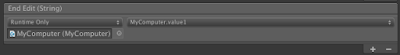Value1 Field 輸入的字串傳給 MyComputer 的 value1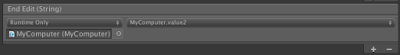Value2 Field 輸入的字串傳給 MyComputer 的 value2Button + 的 On Click 事件執行 MyComputer 的 Add()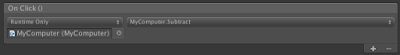Button - 的 On Click 事件執行 MyComputer 的 Subtract()Button x 的 On Click 事件執行 MyComputer 的 Multiply()Button / 的 On Click 事件執行 MyComputer 的 Divide()每個計算功能事件讓 UI 變更運算符號以及顯示計算結果計算結果的畫面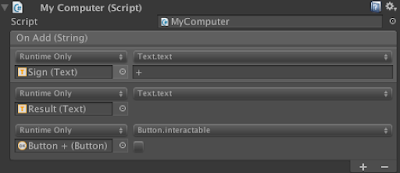計算結果之後關閉按鈕。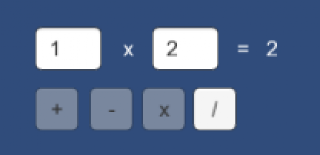計算過的按鈕都關閉了。

[SerializeField]
private UnityEvent onResetStatus;

public void ResetStatus(){

this.onResetStatus.Invoke();
}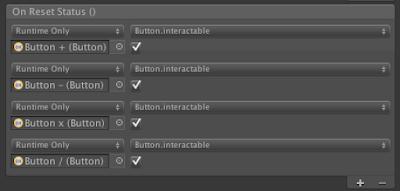狀態重置時，再次啟用按鈕。End Edit 事件指定執行 MyComputer 的 ResetStatus()。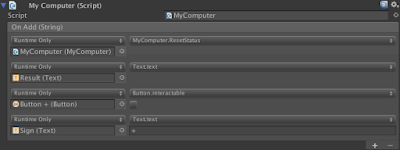更改為先執行狀態重置之後，才去執行其他行為。

using UnityEngine;
using UnityEngine.Events;

public class MyComputer : MonoBehaviour {

private float _value1;
private float _value2;

[SerializeField]
[SerializeField]
private PassString onSubtract;
[SerializeField]
private PassString onMultiply;
[SerializeField]
private PassString onDivide;
[SerializeField]
private UnityEvent onResetStatus;

public string value1{
set{
float.TryParse(value , out this._value1);
}
}

public string value2{
set{
float.TryParse(value , out this._value2);
}
}

}

public void Subtract(){

this.onSubtract.Invoke((this._value1 - this._value2).ToString());
}

public void Multiply(){

this.onMultiply.Invoke((this._value1 * this._value2).ToString());
}

public void Divide(){
if(this._value2 == 0) return;
this.onDivide.Invoke((this._value1 / this._value2).ToString());
}

public void ResetStatus(){

this.onResetStatus.Invoke();
}
}

## 2. Sphere Control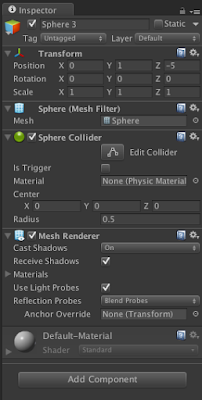原生物件球體的 Componet。

• 球體可以被點擊觸發。
• 球體可以彈跳起來。
• 球體可以變色。

using UnityEngine;
using UnityEngine.Events;

public class SphereTouch : MonoBehaviour {

[SerializeField]
private UnityEvent onTouch;

public void DoTouch(){

this.onTouch.Invoke();
}

void OnMouseDown(){

this.DoTouch();
}
}

[SerializeField]
private float hight = 1;
[SerializeField]
private float speed = 5;

private enum Status{

None,
Moving
}

private Status _status = Status.None;

private IEnumerator Move(Vector3 source , Vector3 target){

float t = 0;

while(t < 1){

transform.position = Vector3.Lerp(source , target , t);
t += Time.deltaTime * this.speed;

yield return null;
}

transform.position = target;
}

Vector3.Lerp 的 t 是介於 0 到 1 的值，我們可以把它當作是起點到終點的進度位置來看待，0 是起點，1 是終點。因為實際執行時每次刷新畫面的時間都不一樣，所以，我們不能讓 t 隨著時間的推移增加固定的值，而應該要為我們預計增加的值（即是速度）乘上 Time.deltaTime，於是，我們在這裡透過 yield return null 讓 while 迴圈每經過一個 frame 才執行一次，當 t 超過 1 的時候，表示已經到達終點，即可結束迴圈。

private IEnumerator DoJump(){

this._status = Status.Moving;

Vector3 source = transform.position;
Vector3 target = source;
target.y += this.hight;

yield return StartCoroutine(this.Move(source , target));
yield return StartCoroutine(this.Move(target , source));

this._status = Status.None;
}

public void Jump(){

if(this._status == Status.None) StartCoroutine(this.DoJump());
}

using UnityEngine;
using System.Collections;

public class SphereJump : MonoBehaviour {

private enum Status{

None,
Moving
}

[SerializeField]
private float hight = 1;
[SerializeField]
private float speed = 5;

private Status _status = Status.None;

public void Jump(){

if(this._status == Status.None) StartCoroutine(this.DoJump());
}

private IEnumerator Move(Vector3 source , Vector3 target){

float t = 0;

while(t < 1){

transform.position = Vector3.Lerp(source , target , t);
t += Time.deltaTime * this.speed;

yield return null;
}

transform.position = target;
}

private IEnumerator DoJump(){

this._status = Status.Moving;

Vector3 source = transform.position;
Vector3 target = source;
target.y += this.hight;

yield return StartCoroutine(this.Move(source , target));
yield return StartCoroutine(this.Move(target , source));

this._status = Status.None;
}
}

[System.Serializable]
public class PassColor : UnityEvent<Color>{}

private Material _material;
private Color _color;

[SerializeField]
private Color color = Color.white;

void Awake(){

this._material = GetComponent<Renderer>().material;
this.DefaultColor();
}

public void DefaultColor(){

this._material.color = this.color;
this._color = this.color;
}

[SerializeField]
private PassColor onChangeColor;

void Awake(){

this._material = GetComponent<Renderer>().material;
this.DefaultColor();
}

public void DefaultColor(){

this._material.color = this.color;
this._color = this.color;
}

public void Discolor(Color color){

this._material.color = color;
this._color = color;
this.onChangeColor.Invoke(color);
}

public void RandomColor(){

this.Discolor(new Color(Random.value , Random.value , Random.value));
}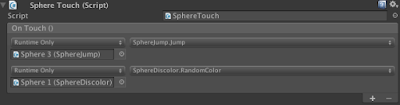第 2 顆球設置被點擊時，第三顆球跳起來，第一顆球隨機改變顏色。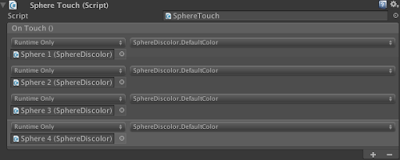第五顆球被點擊時，前四顆球改變成初始顏色。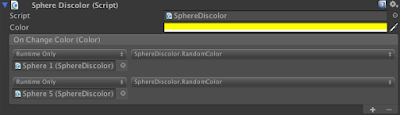第二顆球變色的時候，讓第一顆球和第五顆球改變為隨機的顏色。

public class PassHolder {

public object value{set; private get;}

public T GetValue<T>(){

if(this.value == null) return default(T);

return (T)this.value;
}
}

[System.Serializable]
public class PassColorReturn : UnityEvent<Color , PassHolder>{}

[SerializeField]
private PassColorReturn onSwapColor;

public void SwapColor(){

PassHolder holder = new PassHolder();
this.onSwapColor.Invoke(this._color , holder);
this.Discolor(holder.GetValue<Color>());
}

public void Discolor(Color color , PassHolder holder){

holder.value = this._color;
this.Discolor(color);
}當第四顆球被點擊時，使第五顆球跳起來、第三顆球改變顏色、要求自己執行交換顏色並指明與第一顆球交換顏色。

https://onedrive.live.com/redir?resid=D13D8A29206AFE69!263&authkey=!AMLBuBYFzZsVtOg&ithint=file%2czip